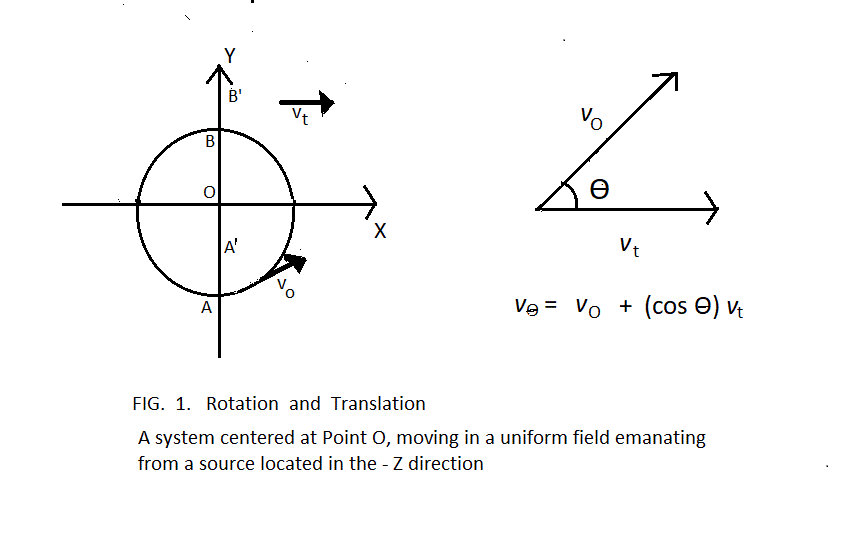Speculations Toward a More Unified Concept of FieldConsider a system rotating in the x-y plane and having translational motion in the + x direction. Such a system is depicted by Figure 1. This paper ... um, perhaps this should be called a thingy ... attempts to reasonably justify forces observed when appropriate systems are seen in a gravitational field or in an electric field.In the case that the system is a rigid object moving in a uniform gravitational field, each particle of matter in the object must have a fixed position relative to all other particles of matter in the object. The required fixed positions make coupling of translation with rotation impossible. Coupling of translation with rotation would cause immediate violation in the constancy of the angular momentum of the particle of matter. Translation and rotation simply remain separate. The result is well known “gyroscopic motion,” with rotation in the x-y plane simply remaining constant, and “projectile motion” in which the object as a whole follows a parabolic trajectory in the x-z plane. For a more detailed discussion, consult any introductory textbook in general physics or a website such as web.mit.edu/8.01t/www/materials/modules/chapter21.pdf.The case is somewhat more complex if the system comprises electric charge moving in a uniform electric field. Electric charge seems to behave more like a compressible fluid than like a rigid object. To conserve the angular momentum of a given bit of charge, that bit of charge must follow an elliptical path, with a minimum radius at Point A in figure 1 and a maximum radius at Point B’ in figure 1. The bit of charge would then follow an elliptical path to again have a minimum radius at Point A’. This requires that a constant force in the plane of rotation be applied in the + y direction (or - y direction for rotation in the opposite direction), to each bit of charge. Since the same constant force must be applied to each bit of charge in the system, the force applies to the system as a whole. With the system as a whole having constant translational velocity, application of a constant perpendicular force causes the system as a whole to move in a circular path in the x-y plane.The key difference between the rigid object and the system of electric charge is that translation cannot couple with rotation in determining the angular momentum of particles in the rigid object, but it can couple with rotation in the case of electric charge. The horizontal force that results from coupling in the case of electric charge might be called something like “field cross wind.” In the case that motion of a system sensitive to gravitation allows translation to couple with rotation, gravitational cross wind might be observable.